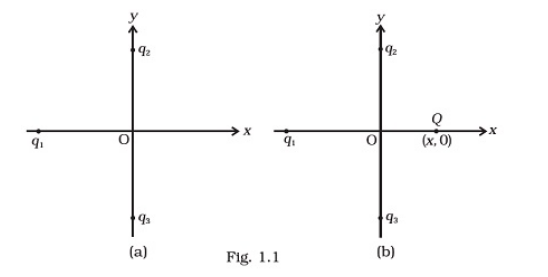Deepak Scored 45->99%ile with Bounce Back Crack Course. You can do it too!

# In figure two positive charges q2 and q3 fixed along the y-axis,

Question:

In figure two positive charges q2 and q3 fixed along the y-axis, exert a net electric force in the + x-direction on a charge q1, fixed along the x-axis. If a positive charge Q is added at (x, 0), the force on q1,(a) shall increase along the positive x-axis

(b) shall decrease along the positive x-axis

(c) shall point along the negative x-axis

(d) shall increase but the direction changes because of the intersection of Q with qand q3

Solution:

Net force on charge q1, by other charges q2 and q3 is along the + x-direction, so nature of force between q1 & q2 and q1 & q3 is attractive. This is possible when charge q1 is negative.

Now, if a positive charge is placed at (x, 0), then, the force on q1 shall increase. The direction will be along positive x axis.